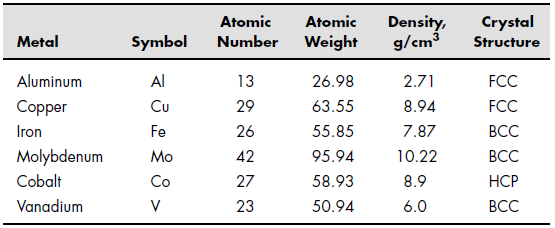# Lesson 10: Other Kinds of Arrays

## Objectives

• Learn the different kinds of data used in MATLAB.

## Background

Data Type

MATLAB provides 15 fundamental data type. Every data type stores data that is in the form of a matrix or array. You can watch Introducing MATLAB Fundamental Classes (Data Types).

## Questions

Number Data Types

1. Define an array of the first 10 integers, using the int8 type designation. Use these integers to calculate the first 10 terms in the harmonic series. The harmonic series:
$\frac{1}{1} + \frac{1}{2} + \frac{1}{3} + \frac{1}{4} + \cdots + \frac{1}{n} + \cdots$
2. Explain why it is better to allow MATLAB to default to double-precision floating-point number representations for most engineering calculations than to specify single and integer types.

Character Data

1. Sometimes it is confusing to realize that numbers can be represented as both numeric data and character data. Use MATLAB to express the number 85 as a character array.
1. How many elements are in this array?
2. What is the numeric equivalent of the character 8?
3. What is the numeric equivalent of the character 5?

Character Arrays

1. Create a two-dimensional array called birthdays to represent the birthday of each person. For example, your array might look something like this:
birthdays=
6  11  1983
3  11  1985
6  29  1986
12  12  1984
12  11  1987
1. Use the num2str function to convert birthdays to a character array.
2. Use the disp function to display a table of names and birthdays.

Cell Arrays

1. Create a cell array called sample_cell to store the following individual arrays:
$A = \left[ {\begin{array}{*{20}{c}}1&3&5\\3&9&2\\{11}&8&2\end{array}} \right]$ (a double-precision floating-point array)
$B = \left[ {\begin{array}{*{20}{c}}{fred}&{ralph}\\{ken}&{susan}\end{array}} \right]$ (a padded character array)
$C = \left[ {\begin{array}{*{20}{c}}4\\6\\3\\1\end{array}} \right]$ (an int8 integer array)
1. Extract array A from sample_cell.
2. Extract the information in array C , row 3, from sample_cell.
3. Extract the name "fred" from sample_cell . Remember that the name fred is a 1×4 array, not a single entity.

Structure Arrays

1. Consider the following information about metals:1. Create the following arrays:
• Store the name of each metal into an individual character array, and store all these character arrays into a cell array.
• Store the symbol for all these metals into a single padded character array.
• Store the atomic number into an int8 integer array.
• Store the atomic weight into a double-precision numeric array.
• Store the density into a single-precision numeric array.Store the structure into a single padded character array.
2. Group the arrays you created in part (a) into a single structure array.
3. Extract the following information from your structure  array:
• Find the name, atomic weight, and structure of the fourth element in the list.
• Find the names of all the elements stored in the array.
• Find the average atomic weight of the elements in the table. (Remember, you need to extract the information to use in your calculation from the cell array.)
• Find the element with the maximum atomic weight.